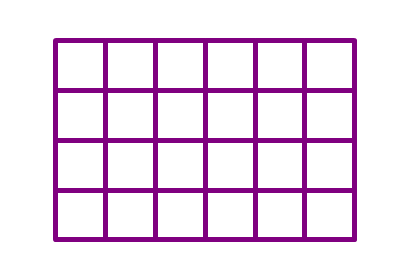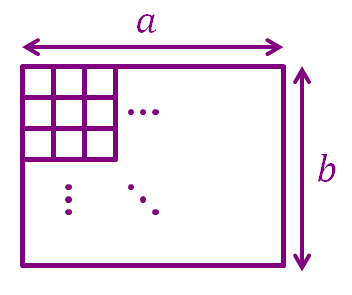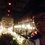# How To Count Quadrilaterals!

Hello again fellow quadrilateral enthusiasts!

Today, we're here to learn how to count quadrilaterals, so sit back, relax, and go grab a cup of coffee if you want to (or tea, if you prefer).

Just in case you don't know, quadrilateral is a polygon that has 4 sides.

Again let's start off with an example:

How many quadrilaterals are there in the $6\times4$ grid below?Of course we could go old school and count them one by one, but we're gonna go the smarter way.

To construct a quadrilateral, we need two horizontal lines, and two vertical lines.

In the $6\times4$ grid above, there are 7 vertical lines and 5 horizontal lines, we choose 2 from each of them.

Hence, the total number of quadrilaterals in a $6\times4$ grid is ${7\choose 2} \times {5\choose 2} =210$

Now, generally, how do we count the number of quadrilaterals in an $a\times b$ grid (where $a$ is the width of the grid and $b$ is the height of the grid)?Similarly, to construct a quadrilateral, we need two horizontal lines and two vertical lines.

In an $a\times b$ grid, there are $a+1$ vertical lines and $b+1$ horizontal lines, we choose two from each of them.

So, the number of quadrilaterals in an $a\times b$ grid is ${a+1\choose 2}\times{b+1\choose 2}=\frac{ab(a+1)(b+1)}{4}$

The number of quadrilaterals in an $a\times b$ grid (where $a$ is the width of the grid, $b$ is the height of the grid) is $\frac{ab(a+1)(b+1)}{4}$

##### This is one part of Quadrilatorics.Note by Kenneth Tan
5 years, 2 months ago

This discussion board is a place to discuss our Daily Challenges and the math and science related to those challenges. Explanations are more than just a solution — they should explain the steps and thinking strategies that you used to obtain the solution. Comments should further the discussion of math and science.

When posting on Brilliant:

• Use the emojis to react to an explanation, whether you're congratulating a job well done , or just really confused .
• Ask specific questions about the challenge or the steps in somebody's explanation. Well-posed questions can add a lot to the discussion, but posting "I don't understand!" doesn't help anyone.
• Try to contribute something new to the discussion, whether it is an extension, generalization or other idea related to the challenge.
• Stay on topic — we're all here to learn more about math and science, not to hear about your favorite get-rich-quick scheme or current world events.

MarkdownAppears as
*italics* or _italics_ italics
**bold** or __bold__ bold
- bulleted- list
• bulleted
• list
1. numbered2. list
1. numbered
2. list
Note: you must add a full line of space before and after lists for them to show up correctly
paragraph 1paragraph 2

paragraph 1

paragraph 2

[example link](https://brilliant.org)example link
> This is a quote
This is a quote
    # I indented these lines
# 4 spaces, and now they show
# up as a code block.

print "hello world"
# I indented these lines
# 4 spaces, and now they show
# up as a code block.

print "hello world"
MathAppears as
Remember to wrap math in $$ ... $$ or $ ... $ to ensure proper formatting.
2 \times 3 $2 \times 3$
2^{34} $2^{34}$
a_{i-1} $a_{i-1}$
\frac{2}{3} $\frac{2}{3}$
\sqrt{2} $\sqrt{2}$
\sum_{i=1}^3 $\sum_{i=1}^3$
\sin \theta $\sin \theta$
\boxed{123} $\boxed{123}$

## Comments

Sort by:

Top Newest

A complex problem has been beautifully simplified. Thanks a lot.

- 5 years, 1 month ago

Log in to reply

Pro.. .

- 4 years, 11 months ago

Log in to reply

I beg to disagree... What if the sides of the quadrilateral are not parallel to the grid?

You cannot count them using this method...

In this method, you can only count squares and rectangles

- 2 years, 10 months ago

Log in to reply

×

Problem Loading...

Note Loading...

Set Loading...